# Ex 9.4 NCERT Solutions- Areas of Parallelograms and Triangles Notes | Study Mathematics (Maths) Class 9 - Class 9

## Class 9: Ex 9.4 NCERT Solutions- Areas of Parallelograms and Triangles Notes | Study Mathematics (Maths) Class 9 - Class 9

The document Ex 9.4 NCERT Solutions- Areas of Parallelograms and Triangles Notes | Study Mathematics (Maths) Class 9 - Class 9 is a part of the Class 9 Course Mathematics (Maths) Class 9.
All you need of Class 9 at this link: Class 9

Question 1. Parallelogram ABCD and rectangle ABEF are on the same base AB have equal areas. Show that the perimeter of the parallelogram is greater than that of the rectangle.
Solution:
We have a parallelogram ABCD and rectangle ABEF such that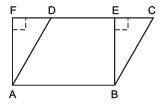ar (parallelogram ABCD) = ar (rectangle ABEF)
∵ AB = CD [∵ Opposite sides of a parallelogram are equal]
and AB = EF [∵ Opposite sides of a rectangle are equal]
⇒ CD = EF ⇒ AB + CD = AB + EF                …(1)
∵ BE < BC
and AF < AD         [∵ In a right triangle, hypotenuse is the longest side.]
∴ BC > BE and AD > AF ⇒ (BC + AD) > (BE + AF)               …(2)
Adding (1) and (2), we have
(AB + CD) + (BC + AD) > (AB + EF) + (BE + AF)
⇒ (AB + BC + CD + DA) > (AB + BE + EF + FA)
⇒ [Perimeter of parallelogram ABCD] > [Perimeter of rectangle ABEF]

Question 2. In the figure, D and E are two points on BC such that BD = DE = EC. Show that ar (ABD) = ar (ADE) = ar (AEC) Can you now, answer the question that you have left in the ‘Introduction’ of this chapter, whether the field of Budhia has been actually divided into three parts of equal area?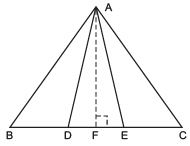Remark Note that by taking BD = DE = EC, the triangle ABC is divided into three triangles ABD, ADE and AEC of equal areas. In the same way, by dividing BC into n equal parts and joining the points of division so obtained to the opposite vertex of BC, you can divide DABC into n triangles of equal areas.
Solution:
Let us draw AF, perpendicular to BC such that AF is the height of ΔABD, ΔADE and ΔAEC.

ar (ΔABD) = (1/2) x BD x AF

[∵ Area of a triangle = (1/2) x base x height]
Similarly, ar (ΔADE) = (1/2) x DE x AF
ar (ΔAEC) = (1/2) x EC x AF
Since, BD = DE = EC

∴ ((1/2) x BD x AF) = ((1/2) x DE x AF) = ((1/2) x EC x AF)

⇒ ar (ΔABD) = ar (ΔADE) = ar (ΔAEC) ,

Yes, the altitudes (heights) of all the triangles are same. Therefore, Budhia can use the above result in dividing her land in three equal parts.

Question 3. In the figure, ABCD, DCFE and ABFE are parallelograms. Show that ar(ADE) = ar (BCF).
Solution:
We have parallelograms ABCD, DCFE and ABFE. ∵ ABCD is a parallelogram [given]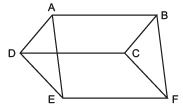∴ Its opposite sides are parallel and equal. ⇒ AD = BC             …(1)
and AB || DC               …(2)
Since, DCFE is a parallelogram                          [Given]
∴ DC || EF              …(3)
From (2) and (3), we have AB || EF
Now, DADE and DBCF are on equal bases,
[from (1)] and between the same parallels,
i.e.  AB || EF

Question 4. In the figure ABCD is a parallelogram and BC is produced to a point Q such that AD = CQ. If AQ intersect DC at P, show that ar (BPC) = ar (DPQ).
Hint: Join AC.
Solution:
We have a parallelogram ABCD and AD = CQ.
Let us join AC. We know that triangles on the same base and between the same parallels are equal in area. Since ΔQAC and ΔQDC are on the same base QC and between the same parallels AD and BQ.
∴ ar (ΔQAC) = ar (ΔQDC) Subtracting ar (ΔQPC) from both sides, we have
ar (ΔQAC) ∠ ar (ΔQPC) = ar (ΔQDC) ∠ ar (ΔQPC)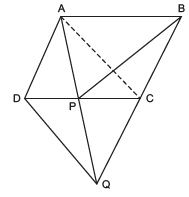⇒ ar (ΔPAC) = ar (ΔQDP)                    ...(1)
Since, ΔPAC ad ΔPBC are on the same base PC and between the same parallels AB and CD.
∴ ar (ΔPAC) = ar (ΔPBC)                      …(2)
From (1) and (2), we get ar (ΔPBC) = ar (ΔQDP)
i.e. ar (ΔPBC) = ar (ΔDPQ)

Question 5. Diagonals AC and BD of a quadrilateral ABCD intersect each other at P. Show that ar (APB) x ar (CPD) = ar (APD) x ar (BPC).
Hint: From A and C, draw perpendiculars to BD.
Solution: We have a quadrilateral ABCD such that its diagonals AC and BD intersect at P.
Let us draw AM ⊥ BD and CN ⊥ BD.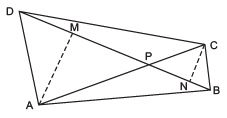Now, ar (ΔAPB) = (1/2) x BP x AM
ar (ΔCPD) =  (1/2) x DP x CN
∴ ar (ΔAPB) x ar (ΔCPD) = ((1/2) x BP x AM) x ((1/2) x DP x CN)
(1/4) x BP x DP x AM x CN                      …(1)
Similarly,
ar Δ(APD) x ar (ΔBPC) = (1/4)x BP x DP x AM x CN                       …(2)
From (1) and (2), we get ar (ΔAPB) x ar (ΔCPD) = ar (ΔAPD) x ar (ΔBPC).

Question 6. P and Q are respectively the mid-points of sides AB and BC of a triangle ABC and R is the mid-point of AP, show that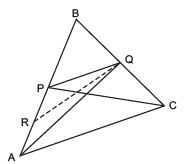(i) ar (∆ PRQ) = (1/2)ar (∆ ARC)

(ii) ar (∆ RQC) = (3/8)ar (∆ ABC)

(iii) ar (∆ PBQ) = ar (∆ ARC) Solution: We have a DABC such that ‘P’ is the mid-point of AB and ‘Q’ is the mid-point of BC. Also, ‘R’ is the mid-point of AP. Let us join AQ and PC.

(i) To prove that ar (ΔPRQ) = (1/2)ar(ΔARC)
In ΔAPQ, R is the mid-point of AP.                      [Given]
Let us join RQ.
∵ RQ is a median of ΔAPQ.
∴ ar (ΔPRQ) = (1/2)ar (ΔAPQ)                     …(1)
∵ QP is a median of ΔABQ.                    [∵ P is the mid-point of AB]
∴ ar (ΔAPQ) = (1/2)ar (ΔABQ)                 …(2)
From (1) and (2), we get ar (ΔPRQ) = (1/2)x (1/2) ar (ΔABQ)
= (1/4) ar (ΔABQ)
= (1/4) x (1/2) ar (ΔABC)                   [ ∵ AQ is a median of ΔABC]
=  (1/8)(ar ABC) … (3)
Now, ar (ΔARC) = (1/2)ar (ΔAPC)              [∵ CR is a median of ΔAPC]
= (1/2)x (1/2)ar (ΔABC)                   [∵ CP is a median of ΔABC]
= (1/4)ar (ΔABC)                                ... (4)
Now from (3) and (4), we get ar (ΔPRQ) = (1/8)ar (ΔABC)

= (1/2) x (1/4)ar ABC)
= (1/2)ar (ΔARC)
Thus, ar (ΔPRQ) = (1/2) ar (ΔARC)

(ii) To prove that ar (ΔRQC) = (3/8)ar (ΔABC)
In ΔRBC, RQ is a median. ∴
ar (ΔRQC) = ar (ΔRBQ) = ar (ΔPRQ) + ar (ΔBPQ)
= (1/8)ar (ΔABC) + (1/2) ar (ΔPBC)                   [Proved in (i)]
= (1/8)ar (ΔABC) + (1/2) . (1/2) (ΔABC)]
[∵ P is the mid-point of AB]
= (1/8) ar (ΔABC) + (1/4)ar (ΔABC)]
= ( (1/4) + (1/8) ) ar (ΔABC)
= (3/8)ar (ΔABC)

(iii) To prove that ar (ΔPBQ)
= ar (ΔARC)
∵ PQ is a median of ΔABQ.
∴ ar (ΔPBQ) = (1/2)(ΔABQ)
= (1/2) x (1/2)ar (ΔABC)              [∵ AQ is a median of D ABC]
= (1/4)ar (ΔABC)
= ar (ΔARC) [using (4)]
Thus, ar (ΔPBQ) = ar (ΔARC)

Question 7. In the figure, ABC is a right triangle right angled at A. BCED, ACFG and ABMN are squares on the sides BC, CA and AB respectively. Line segment AX ⊥ DE meets BC at Y. Show that: (i) ΔMBC ≌ ΔABD (ii) ar (BYXD) = 2ar (∆ MBC) (iii) ar (BYXD) = ar (ABMN) (iv) ΔFCB ≌ ΔACE (v) ar (CYXE) = 2ar (∆ FCB) (vi) ar (CYXE) = ar (ACFG) (vii) ar (BCED) = ar (ABMN) + ar (ACFG)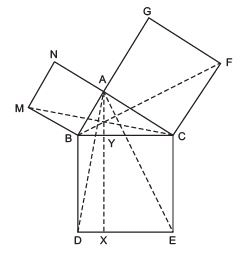Note:  Result (vii) is the famous Theorem of Pythagoras. You shall learn a simpler proof of this theorem in Class X.
Solution:
We have a right DABC such that BCED, ACFG and ABMN are squares on its sides BC, CA and AB respectively.
Line segment AX ⊥ DE is also drawn such that it meets BC in Y.

(i) To prove that ΔMBC ≌ ΔABD In DABD and DMBC, we have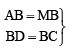[Sides of a square]
∠CBD = ∠MBA [Each = 90º]
∴ ∠CBD + ∠ABC = ∠MBA + ∠ ABC
or ∠MBC = ∠ABD
∴ ΔMBC ≌ ΔABD
(ii) To prove that: ar (BYXD) = 2 ar (∆ MBC)
Since parallelogram BYXD and DABD are on the same base BD and between the same parallels BD and AX.

ar (ΔABD) = (1/2) ar (parallelogram BYXD)
But ar (ΔABD) = ar (ΔMBC) [∵ Congruent triangles have equal areas]
⇒ ar (ΔMBC) = (1/2) ar (parallelogram BYXD)
or 2ar (ΔMBC) = ar (BYXD) Thus, ar (BYXD)
= 2ar (ΔMBC)

(iii) To prove that ar (BYXD) = ar (ABMN)
∵ ar (BYXD) = 2ar (ΔMBC)                      [from (ii)]                    …(1)

and ar (ABMN) = 2ar (MBC)               ...(2)           [∵ ABMN and DMBC are on the same base MB and between the same parallels MB and NC]
∴ From (1)  and (2), we have ar (BYXD) = ar (ABMN)

(iv) ΔFCB ≌ ΔACE
In ΔFCB and ΔACE, F C = AC               [Sides of a square]
CB = CE                         [Sides of a square]
∠FCA = ∠BCE
or ∠FCA + ∠ACB = ∠BCE + ∠ACB
⇒∠FCB = ∠ACE
⇒ΔFCB ≌ ΔACE           [SAS rule]

(v) To prove that ar (CYXE) = 2ar (FCB)
Since (CYXE) and (ΔACE) are on the same base CE and between the same parallels CE and AX.
∴ ar (CYXE) = 2ar (ΔACE)
But ΔACE ≌ ΔFCB
Since, congruent triangles are equal in areas.
∴ ar (CYXE) = 2ar(ΔFCB)

(vi) To prove ar(CYXE) = ar (ACFG)
Since, ar (CYXE) = 2ar (ΔFCB)               [from (v)]              ...(3)
Also, (ACFG) and ΔFCB are on the same base FC and between the same parallels FC and BG.
⇒ ar (ACFG) = 2ar (ΔFCB)                      …(4)
From (3) and (4), we get ar (CYXE) = ar (ACFG)                     …(5)

(vii ) To prove that ar (BCED) = ar (ABMN) + ar (ACFG)
We have ar (BCED) = ar (CYXE) + ar (BYXD) = ar (CYXE) + ar (ABMN)                         [from (iii)]
Now, ar (BCED) = ar (ABMN) + ar (CYXE).
Thus, ar (BCED) = ar (ABMN) + ar (ACFG)                 [from (5)

The document Ex 9.4 NCERT Solutions- Areas of Parallelograms and Triangles Notes | Study Mathematics (Maths) Class 9 - Class 9 is a part of the Class 9 Course Mathematics (Maths) Class 9.
All you need of Class 9 at this link: Class 9Use Code STAYHOME200 and get INR 200 additional OFF

## Mathematics (Maths) Class 9

73 videos|351 docs|110 tests

Track your progress, build streaks, highlight & save important lessons and more!

,

,

,

,

,

,

,

,

,

,

,

,

,

,

,

,

,

,

,

,

,

;